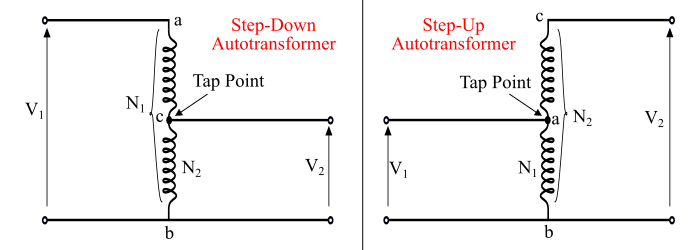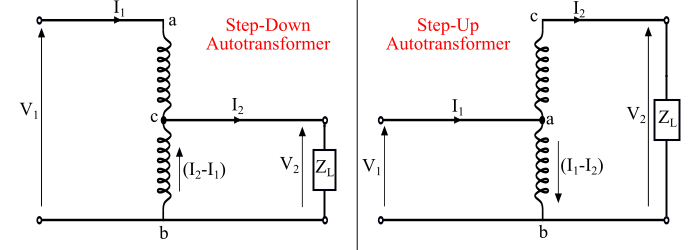# What is an Autotransformer?

An autotransformer is a one winding transformer in which a part of the winding is common to both primary and secondary windings.

An autotransformer has a single continuous winding with a tap point between the primary and secondary windings. The tap point can be adjusted to obtain the desired output voltage, hence this is an obvious advantage of the autotransformer. The main disadvantage of an autotransformer is that the secondary winding is not electrically isolated from the primary.

## Theory of Autotransformer

### Case 1 – Autotransformer on No-Load

The connection diagram of an unloaded autotransformer (step-down and step-up) is shown in the figure. In this, the winding 'ab' is called primary winding having N1 turns and the winding 'bc' is called secondary winding having N2 turns.Here it can be noted that the primary and secondary windings are connected magnetically as well as electrically. Thus, the power from the primary winding is transferred to the secondary winding magnetically as well as conductively.

### Case 2 – Autotransformer on Load

When the load is connected to the secondary of an autotransformer, then it is said to be loaded. The figure shows the circuit diagram of a loaded autotransformer (step-down and step-up).In which the current I1 is the primary input current and the current I2 is the secondary output current or load current. The current in common portion of the primary and secondary windings is the difference of the currents I1 and I2, regardless of whether the autotransformer is stepdown or step-up.

For the step-down autotransformer the current I2 > I1, thus the current flowing in the common portion is

$$\mathrm{Current\:in\:common\:portion = 𝐼_{2} − 𝐼_{1}}$$

For the step-up autotransformer the current I2 < I1, thus the current in the common portion is

$$\mathrm{Current\:in\:common\:portion = 𝐼_{1} − 𝐼_{2}}$$

The advantages of an autotransformer are given as follows −

• An autotransformer has smaller core and copper losses and hence higher efficiency as compared to an ordinary 2-winding transformer.

• Autotransformer requires less conductor material as compared to the 2-winding transformer.

• It has better voltage regulation due to reduced voltage drops in resistance and leakage reactance.

• It has smaller size and cheaper than a 2-winding transformer.

• It requires smaller excitation current.

• An autotransformer can produce variable output voltage.

The disadvantages of an autotransformer are given as follows −

• There is a direct connection between the primary and secondary sides. In case of an open circuit in the common portion bc of the windings, the full primary voltage would be applied to the load on the secondary side. This may damage the equipment connected to the secondary side.

• An autotransformer has reduced internal impedance as compared to a 2-winding transformer which results in a larger short circuit current.

• The autotransformer is not electrically isolated, thus cannot be used to isolate two circuits electrically.

## Applications of Autotransformer

The autotransformer is mainly used in following applications −

• The autotransformers are used for boosting of supply voltage to compensate the voltage drops in distribution systems.

• Autotransformers are used for interconnection of power systems of different voltage levels.

• The autotransformers with tapings are used for starting induction motors and synchronous motors.

• The autotransformers are used as variac (variable AC) in the situations where a continuous variable voltage over a wide range is required.